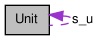# Unit Class Reference [Input File Handling]

Unit conversion utility. More...

`#include <units.h>`

Collaboration diagram for Unit:[legend]

List of all members.

## Public Member Functions

virtual ~Unit ()
Empty Destructor.
doublereal actEnergyToSI (std::string units)
Return the multiplier required to convert an activation energy to SI units.
doublereal toSI (std::string units)
Return the multiplier required to convert a dimensional quantity with units specified by string 'units' to SI units.

## Static Public Member Functions

static Unitunits ()
Initialize the static Unit class.
static void deleteUnit ()
Destroy the static Unit class.

Unit ()

## Private Attributes

std::map< std::string, doublereal > m_u
Map between a string and a units double value.
std::map< std::string, doublereal > m_act_u
Map between a string and a units double value for activation energy units.

## Static Private Attributes

static Units_u = 0
pointer to the single instance of Unit
static boost::mutex units_mutex
Decl for static locker for Units singelton.

## Detailed Description

Unit conversion utility.

Definition at line 33 of file units.h.

## Constructor & Destructor Documentation

 virtual ~Unit ( ) ` [inline, virtual]`

Empty Destructor.

Definition at line 60 of file units.h.

 Unit ( ) ` [inline, private]`

Units class constructor, containing the default mappings between strings and units.

Definition at line 180 of file units.h.

## Member Function Documentation

 doublereal actEnergyToSI ( std::string units ) ` [inline]`

Return the multiplier required to convert an activation energy to SI units.

Parameters:
 units activation energy units

Definition at line 67 of file units.h.

References Unit::m_act_u, and Unit::toSI().

Referenced by Cantera::actEnergyToSI().

 static void deleteUnit ( ) ` [inline, static]`

Destroy the static Unit class.

Note this can't be done in a destructor.

Definition at line 49 of file units.h.

References Unit::s_u, and Unit::units_mutex.

Referenced by Cantera::appdelete().

 doublereal toSI ( std::string units ) ` [inline]`

Return the multiplier required to convert a dimensional quantity with units specified by string 'units' to SI units.

The list of recognized units is storred as a stl map <string, doublereal>called m_u[] and m_act_u for activity coefficients. These maps are initialized with likely values.

Parameters:
 units String containing the units description

Definition at line 85 of file units.h.

References Unit::m_u.

Referenced by Unit::actEnergyToSI(), and Cantera::toSI().

 static Unit* units ( ) ` [inline, static]`

Initialize the static Unit class.

Definition at line 37 of file units.h.

References Unit::s_u, and Unit::units_mutex.

Referenced by Cantera::actEnergyToSI(), Application::Application(), and Cantera::toSI().

## Member Data Documentation

 std::map m_act_u` [private]`

Map between a string and a units double value for activation energy units.

This map maps the dimension string to the units value adjustment. Example

• m_act_u["K"] = GasConstant;

Definition at line 169 of file units.h.

Referenced by Unit::actEnergyToSI(), and Unit::Unit().

 std::map m_u` [private]`

Map between a string and a units double value.

This map maps the dimension string to the units value adjustment. Example

• m_u["m"] = 1.0;
• m_u["cm"] = 0.01;

Definition at line 162 of file units.h.

Referenced by Unit::toSI(), and Unit::Unit().

 Unit * s_u = 0` [static, private]`

pointer to the single instance of Unit

Definition of the static member of the Unit class.

Definition at line 154 of file units.h.

Referenced by Unit::deleteUnit(), and Unit::units().

 boost::mutex units_mutex` [static, private]`

Decl for static locker for Units singelton.

Definition at line 173 of file units.h.

Referenced by Unit::deleteUnit(), and Unit::units().

The documentation for this class was generated from the following files:
Generated by1.6.3